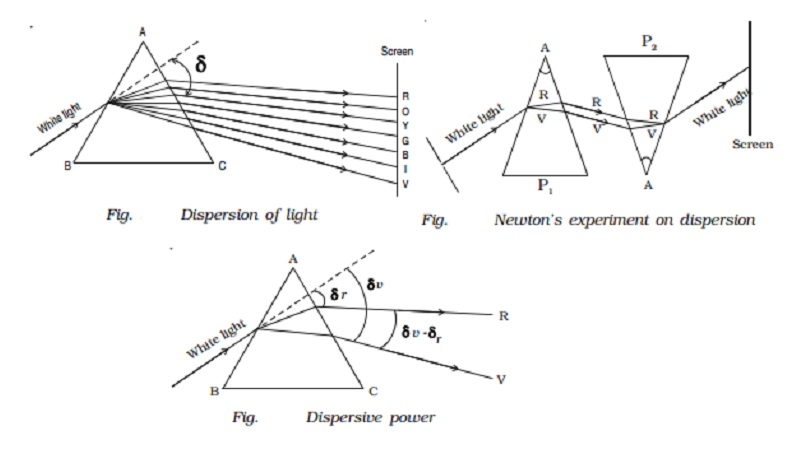Home | | Physics | | Physics | Dispersion of light and Dispersive power

# Dispersion of light and Dispersive powerDispersion is the splitting of white light into its constituent colours. This band of colours of light is called its spectrum.

Dispersion of light

Dispersion is the splitting of white light into its constituent colours. This band of colours of light is called its spectrum.

In the visible region of spectrum, the spectral lines are seen in the order from violet to red. The colours are given by the word VIBGYOR (Violet,Indigo, Blue, Green,Yellow, Orange and Red) (Fig. )The origin of colour after passing through a prism was a matter of much debate in physics. Does the prism itself create colour in some

way or does it only separate the colours already present in white light?

Sir Isaac Newton gave an explanation for this. He placed another similar prism in an inverted position. The emergent beam from the first

prism was made to fall on the second prism (Fig. ). The resulting emergent beam was found to be white light. The first prism separated the white light into its constituent colours, which were then recombined by the inverted prism to give white light. Thus it can be concluded that the prism does not create any colour but it only separates the white light into its constituent colours.Dispersion takes place because the refractive index of the material of the prism is different for different colours (wavelengths). The deviation and hence the refractive index is more for violet rays of light than the corresponding values for red rays of light. Therefore the violet ray travels with a smaller velocity in glass prism than red ray. The deviation and the refractive index of the yellow ray are taken as the mean values. Table 9.2 gives the refractive indices for different wavelength for crown glass and flint glass.

Table List Refractive indices for different wavelengths

Colour         Wave length (nm) Crown glass         Flint glass

Violet 396.9 1.533 1.663

Blue   486.1 1.523 1.639

Yellow         589.3 1.517 1.627

Red    656.3 1.515 1.622The speed of light is independent of wavelength in vacuum. Therefore vacuum is a non-dispersive medium in which all colours travel with the same speed.

Dispersive power

The refractive index of the material of a prism is given by the relation ? = [sin(A+D / 2)] / [sin(A/2)]

Here A is the angle of the prism and D is the angle of minimum deviation.

If the angle of prism is small of the order of 10o, the prism is said to be small angled prism. When rays of light pass through such prisms

the angle of deviation also becomes small.

If A be the refracting angle of a small angled prism and δ the angle of deviation, then the prism formula becomes ? = [sin(A+δ)/2]/sin(A/2)For small angles A and δ,

Sin[(A+δ )/2] = (A+δ )/2 and sin(A/2)=A/2

? = [(A+δ)/2]  / [A/2]

? A = A + δ

δ = (? - 1)A ... (1)

If δv  and δr are the deviations produced for the violet and red rays and ?v and ?r are the corresponding refractive indices of the material of the small angled prism then,

for violet light, δv =(  ? -1  )A  ????..(2)

for red light, δ r = ( ? r  -1  )A   ????..(3)

From equations (2) and (3)

δv - δr = ( ?v - ?r )A             ?????.(4)

δv - δr is called the angular dispersion which is the difference in deviation between the extreme colours (Fig. ).

If δy  and ?y  are the deviation and refractive index respectively for yellow ray (mean wavelength) then,

for yellow light, δ y =( ?y -1) A ... (5)

Dividing equation (4) by (5) we get

δvr / δy  = ( ?v - ?r )/  ( ?y ? 1)

The expression ( δvr)  / (δy) is known as the dispersive power of the material of the prism and is denoted by ω.

ω = ( ?v - ?r )/  ( ?y ? 1)

The dispersive power of the material of a prism is defined as the ratio of angular dispersion for any two wavelengths (colours) to the deviation of mean wavelength.

Study Material, Lecturing Notes, Assignment, Reference, Wiki description explanation, brief detail

Related Topics